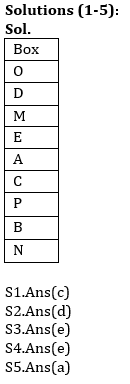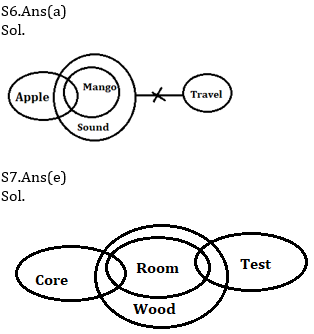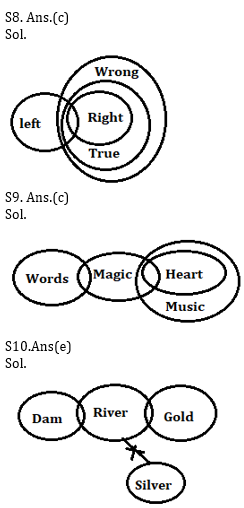Latest Banking jobs   »   Reasoning Ability Quiz For IBPS Clerk/NIACL...

# Reasoning Ability Quiz For IBPS Clerk/NIACL AO Prelims 2021- 4th September

Directions (1-5): Study the information carefully and answer the questions given below.
Nine boxes are placed in a stack one above another. Not more than three boxes placed below box C. Three boxes are placed between box C and box D. Box O is placed just above box D. Box P is placed above box B and below box E. Box N is placed at bottom. Box A is placed above box C and below box E. More than four boxes are placed between box N and box M. Neither box A nor box M is placed at top of stack. Not more than one box is placed between box M and box A.

Q1. Which of the following box is placed just above box C?
(a) Box B
(b) Box P
(c) Box A
(d) Box N
(e) None of these

Q2. Which of the following box is placed just below box P?
(a) Box N
(b) Box C
(c) Box M
(d) Box B
(e) None of these

Q3. How many boxes are placed in between box D and Box B?
(a) One
(b) Four
(c) Two
(d) Three
(e) More than Four

Q4. Four of the following five are alike in certain way based from a group, find the one that does not belong to that group?
(a) E-C
(b) P-N
(c) M-A
(d) O-M
(e) D-P

Q5. Which of the following box is placed at top of stack?
(a) Box O
(b) Box D
(c) Box M
(d) Box P
(e) None of these

Directions (6-10): In each of the questions below are given some statements followed by some Conclusions. You have to take the given statements to be true even, if they seem to be at variance from commonly known facts. Read all the conclusions and then decide which of the given conclusions logically follows from the given statements disregarding commonly known facts.
(a) If only conclusion I follows.
(b) If only conclusion II follows.
(c) If either conclusion I or II follows.
(d) If neither conclusion I nor II follows.
(e) If both conclusions I and II follow.

Q6. Statements:
Some Apple are Mango
All Mango are Sound
No sound is Travel
Conclusion:
I: Some apple are not travel
II: No mango are apple

Q7. Statements:
All room are wood
Some core are room
Some Test are Room
Conclusion:
I: All Test can be wood
II: Some core are wood

Q8. Statements:
Some Left are right
All right are true
All true are wrong
Conclusions:
I. All left are wrong
II. Some left are not wrong

Q9. Statements:
Some words are magic.
Some magic is Heart.
All Heart are Music.
Conclusions:
I. Some words are Music
II. No word is music

Q10. Statements:
Some dam are River
Some River are Gold
No River is Silver
Conclusions:
I: All Dam can be Gold
II: Some Gold are not Silver

Q11. How many pairs of letters are there in the word “Adequate” each of which have as many letters between them in the word as they have between them in the English alphabetical series?
(a) Three
(b) One
(c) Two
(d) More than three
(e) None

Q12. If in the number 63143214, addition of 1 after multiplying by 2 in each of the odd digit and subtract 3 after multiplying by 2 in each of the even digit then, How many digits are repeating in new number ?
(a) Three
(b) One
(c) None
(d) Two
(e) Four

Q13. Which of the following elements should come in a place of ‘?’
JQ4 HS9 FU16 ?
(a) EV36
(b) PK 81
(c) IR 100
(d) DW25
(e) LO 49

Q14. Arun’s ranked 38th from the top and 44th from the bottom in a class. How many students are there in the class?
(a) 82
(b) 77
(c) 81
(d) 79
(e) None of these

Q15. If in the number 867351429, all digits are arranged in increasing order from right to left than how many digit remain same place in the new arrangement?
(a) Three
(b) Four
(c) none
(d) Two
(e) One

SolutionsS11. Ans.(c)
Sol. (de, qt)

S12. Ans.(a)
Sol. Original number- 63143214
Obtained number- 97357135

S13. Ans.(d)

S14. Ans(c)
Sol. Number of students in the class= 38+44-1=81

S15. Ans(a)

Click Here to Register for Bank Exams 2021 Preparation Material#### Congratulations!Download Hindu Review of October 2021: Free PDF